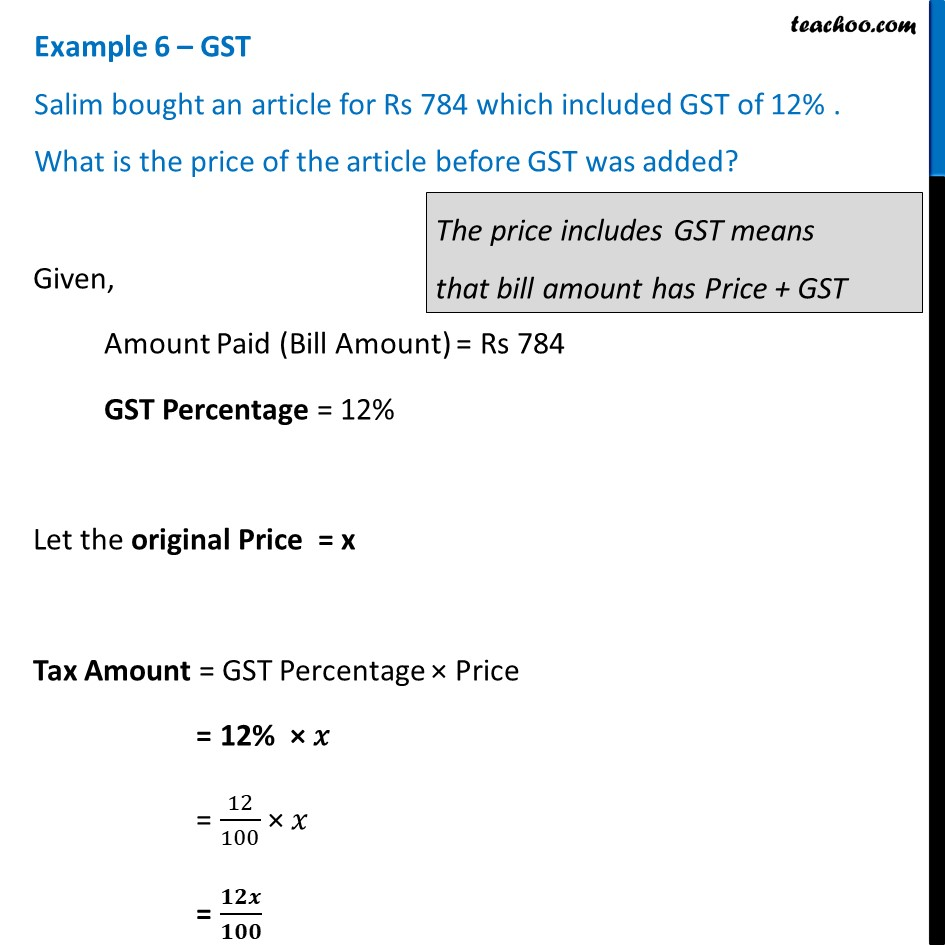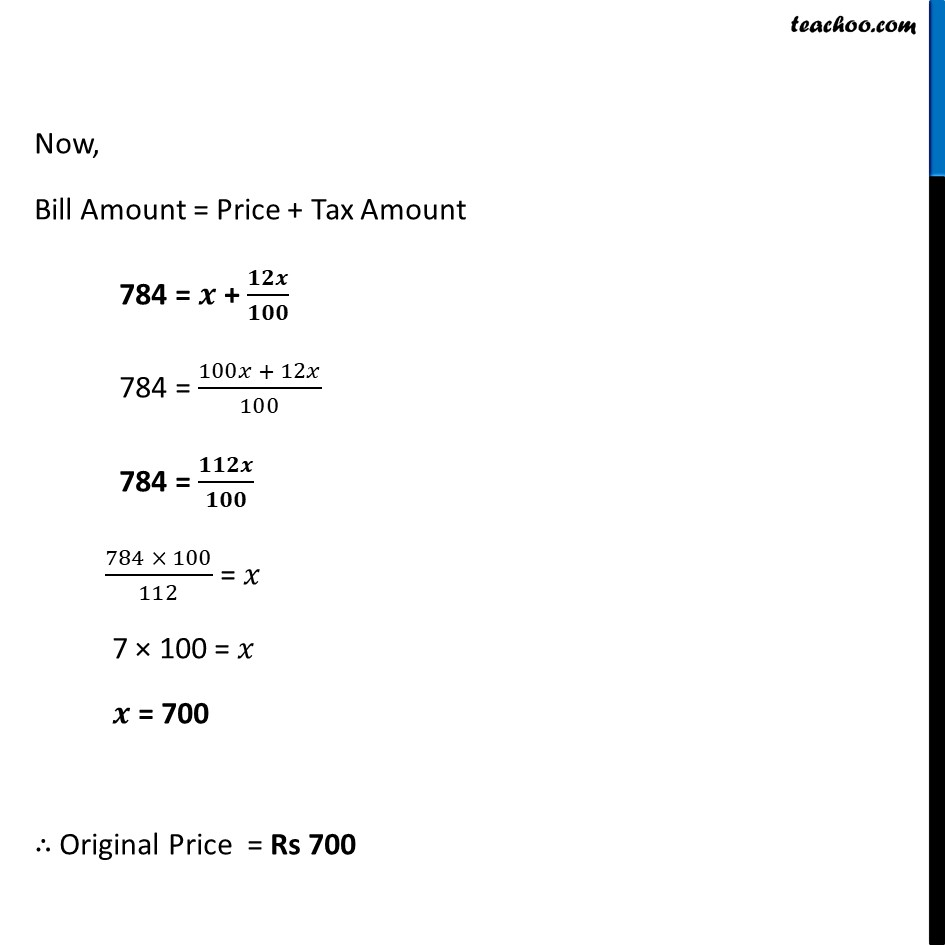Examples

Chapter 7 Class 8 Comparing Quantities
Serial order wiseLearn in your speed, with individual attention - Teachoo Maths 1-on-1 Class

### Transcript

Example 6 – GST ﻿Salim bought an article for Rs 784 which included GST of 12% . What is the price of the article before GST was added? Given, Amount Paid (Bill Amount) = Rs 784 GST Percentage = 12% Let the original Price = x Tax Amount = GST Percentage × Price = 12% × 𝑥 = 12/100 × 𝑥 = 𝟏𝟐𝒙/𝟏𝟎𝟎 Now, Bill Amount = Price + Tax Amount 784 = 𝒙 + 𝟏𝟐𝒙/𝟏𝟎𝟎 784 = (100𝑥 + 12𝑥)/100 784 = 𝟏𝟏𝟐𝒙/𝟏𝟎𝟎 (784 × 100)/112 = 𝑥 7 × 100 = 𝑥 𝒙 = 700 ∴ Original Price = Rs 700